Test: Boundary Layer Theory, Laminar Flow - 2

# Test: Boundary Layer Theory, Laminar Flow - 2

Test Description

## 30 Questions MCQ Test GATE Mechanical (ME) 2023 Mock Test Series | Test: Boundary Layer Theory, Laminar Flow - 2

Test: Boundary Layer Theory, Laminar Flow - 2 for Mechanical Engineering 2022 is part of GATE Mechanical (ME) 2023 Mock Test Series preparation. The Test: Boundary Layer Theory, Laminar Flow - 2 questions and answers have been prepared according to the Mechanical Engineering exam syllabus.The Test: Boundary Layer Theory, Laminar Flow - 2 MCQs are made for Mechanical Engineering 2022 Exam. Find important definitions, questions, notes, meanings, examples, exercises, MCQs and online tests for Test: Boundary Layer Theory, Laminar Flow - 2 below.
Solutions of Test: Boundary Layer Theory, Laminar Flow - 2 questions in English are available as part of our GATE Mechanical (ME) 2023 Mock Test Series for Mechanical Engineering & Test: Boundary Layer Theory, Laminar Flow - 2 solutions in Hindi for GATE Mechanical (ME) 2023 Mock Test Series course. Download more important topics, notes, lectures and mock test series for Mechanical Engineering Exam by signing up for free. Attempt Test: Boundary Layer Theory, Laminar Flow - 2 | 30 questions in 90 minutes | Mock test for Mechanical Engineering preparation | Free important questions MCQ to study GATE Mechanical (ME) 2023 Mock Test Series for Mechanical Engineering Exam | Download free PDF with solutions
 1 Crore+ students have signed up on EduRev. Have you?
Test: Boundary Layer Theory, Laminar Flow - 2 - Question 1

### Which one of the following is the characteristic of a fully developed laminar flow?

Detailed Solution for Test: Boundary Layer Theory, Laminar Flow - 2 - Question 1

Ans. (c)

Test: Boundary Layer Theory, Laminar Flow - 2 - Question 2

### The MINIMUM value of friction factor ‘f’ that can occur in laminar flow through a circular pipe is:

Detailed Solution for Test: Boundary Layer Theory, Laminar Flow - 2 - Question 2

Ans. (b) Friction Factor,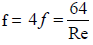Where Max. Re = 2000.

Test: Boundary Layer Theory, Laminar Flow - 2 - Question 3

### A smooth flat plate with a sharp leading edge is placed along a gas stream flowing at U = 10 m/s. The thickness of the boundary layer at section r - s is 10 mm, the breadth of the plate is 1 m (into the paper) and the density of the gas  ρ = 1.0 kg/m3 . Assume that the boundary layer is thin, two-dimensional, and follows a linear velocity distribution, u = U(y/δ), at the section r - s , where y is the height from plate.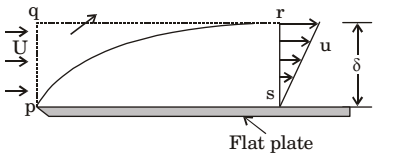The integrated drag force (in N) on the plate, between p - s , is:​​​​

Detailed Solution for Test: Boundary Layer Theory, Laminar Flow - 2 - Question 3

Ans. (c) By momentum equation, we can find drag force.

Test: Boundary Layer Theory, Laminar Flow - 2 - Question 4

In the boundary layer, the flow is:

Detailed Solution for Test: Boundary Layer Theory, Laminar Flow - 2 - Question 4

Ans. (a)

Test: Boundary Layer Theory, Laminar Flow - 2 - Question 5

In the region of the boundary layer nearest to the wall where velocityis not equal to zero, the viscous forces are:

Detailed Solution for Test: Boundary Layer Theory, Laminar Flow - 2 - Question 5

Ans. (c) Reynold’s number = Inertia force / Viscous force and it is more than one therefore the viscous forces are less than  inertial forces.

Test: Boundary Layer Theory, Laminar Flow - 2 - Question 6

The hydrodynamic boundary layer thickness is defined as the distance from the surface where the

Detailed Solution for Test: Boundary Layer Theory, Laminar Flow - 2 - Question 6

Ans. (d)

Test: Boundary Layer Theory, Laminar Flow - 2 - Question 7

Assertion (A): The 'dimples' on a golf ball are intentionally provided.

Reason (R): A turbulent boundary layer, since it has more momentumthan a laminar boundary layer, can better resist an adverse pressure gradient.

Detailed Solution for Test: Boundary Layer Theory, Laminar Flow - 2 - Question 7

Ans. (a)

Test: Boundary Layer Theory, Laminar Flow - 2 - Question 8

During the flow over a circular cylinder, the drag coefficient dropssignificantly at a critical Reynolds number of 2 × 105. This is due to

Detailed Solution for Test: Boundary Layer Theory, Laminar Flow - 2 - Question 8

Ans. (d) The drag co-efficient remains practically constant unit a Reynold’s number of 2 × 105 is reached. At this stage the Cd drops steeply by a factor of 5.
This is due to the fact that the laminar boundary layer turns turbulent and stays unseparated over a longer distance, then reducing the wake considerably.

Test: Boundary Layer Theory, Laminar Flow - 2 - Question 9

What is the commonly used boundary layer control method to prevent separation?

Detailed Solution for Test: Boundary Layer Theory, Laminar Flow - 2 - Question 9

Ans. (d) Following are some of the methods generally adopted to retard separation:
1. Streamlining the body shape.
2. Tripping the boundary layer from laminar to turbulent by provision roughness.
3. Sucking the retarded flow.
4. Injecting high velocity fluid in the boundary  layer.
5. Providing slots near the leading edge.
6. Guidance of flow in a confined passage.
7. Providing a rotating cylinder near the leading edge.
8. Energizing the flow by introducing optimum amount of swirl in the incoming
flow.

Test: Boundary Layer Theory, Laminar Flow - 2 - Question 10

Prandtl number of a flowing fluid greater than unity indicates that hydrodynamic boundary layer thickness is:

Detailed Solution for Test: Boundary Layer Theory, Laminar Flow - 2 - Question 10

Ans. (a)

Test: Boundary Layer Theory, Laminar Flow - 2 - Question 11

Velocity defect in boundary layer theory is defined as

Detailed Solution for Test: Boundary Layer Theory, Laminar Flow - 2 - Question 11

Ans. (b)

Test: Boundary Layer Theory, Laminar Flow - 2 - Question 12

Velocity distribution in a turbulent boundary layer follows

Detailed Solution for Test: Boundary Layer Theory, Laminar Flow - 2 - Question 12

Ans. (a)

Test: Boundary Layer Theory, Laminar Flow - 2 - Question 13

Assertion (A): The thickness of boundary layer cannot be exactlydefined.
Reason (R): The Velocity within the boundary layer approaches theinviscid velocity asymptotically.

Detailed Solution for Test: Boundary Layer Theory, Laminar Flow - 2 - Question 13

Ans. (a)

Test: Boundary Layer Theory, Laminar Flow - 2 - Question 14

The equation of the velocity distribution over a plate is given byu = 2y – y2 where u is the velocity in m/s at a point y meter from theplate measured perpendicularly. Assuming μ = 8.60 poise, the she arstress at a point 15 cm from the boundary is:

Detailed Solution for Test: Boundary Layer Theory, Laminar Flow - 2 - Question 14

Ans. (b)

Test: Boundary Layer Theory, Laminar Flow - 2 - Question 15

How is the displacement thickness in boundary layer analysis defined?

Detailed Solution for Test: Boundary Layer Theory, Laminar Flow - 2 - Question 15

Ans. (c)

Test: Boundary Layer Theory, Laminar Flow - 2 - Question 16

Consider the following statements comparing turbulent boundarylayer with laminar boundary layer:
1. Turbulent boundary layers are thicker than laminar boundary layer
2. Velocity in turbulent boundary layers is more uniform3. In case of a laminar boundary layer, the thickness of the boundarylayer increases more rapidly as the distance from the leading edgeincreases.
4. For the same local Reynolds number. Shear stress at the boundaryis less in the case of turbulent boundary layer.
Of these statements:

Detailed Solution for Test: Boundary Layer Theory, Laminar Flow - 2 - Question 16

Ans. (a)

Test: Boundary Layer Theory, Laminar Flow - 2 - Question 17

Assertion (A): In an ideal fluid, separation from a continuous surfacewould not occur with a positive pressure gradient.
Reason (R): Boundary layer does not exist in ideal fluid.

Detailed Solution for Test: Boundary Layer Theory, Laminar Flow - 2 - Question 17

Ans. (a) In Ideal fluid viscosity is zero so no boundary layer is formed.

Test: Boundary Layer Theory, Laminar Flow - 2 - Question 18

Match List-I (Device) with List-II (Use) and select the correct answer using the codes given below the Lists: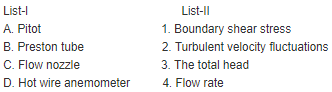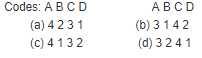Detailed Solution for Test: Boundary Layer Theory, Laminar Flow - 2 - Question 18

Ans. (b)

Test: Boundary Layer Theory, Laminar Flow - 2 - Question 19

Flow separation is caused by

Detailed Solution for Test: Boundary Layer Theory, Laminar Flow - 2 - Question 19

Ans. (c) Separation takes place where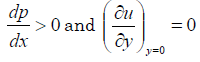Test: Boundary Layer Theory, Laminar Flow - 2 - Question 20

Flow separation is likely to take place when the pressure gradient inthe direction of flow is:

Detailed Solution for Test: Boundary Layer Theory, Laminar Flow - 2 - Question 20

Ans. (b)

Test: Boundary Layer Theory, Laminar Flow - 2 - Question 21

Flow separation is caused by:

Detailed Solution for Test: Boundary Layer Theory, Laminar Flow - 2 - Question 21

Ans. (c) i.e. an adverse pressure gradient.
When the pressure goes increasing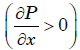in the direction of flow, the pressure force acts against the direction of direction of flow thus retarding the flow. This has an effect of retarding the flow in the boundary layer and hence thickenings the boundary layer more rapidly. This and the boundary shear bring the fluid in the boundary layer to rest and causes back flow. Due to this
the boundary layer no more sticks to the boundary but is shifted away from the
boundary. This phenomenon is called as “Boundary Layer Separation”.

Test: Boundary Layer Theory, Laminar Flow - 2 - Question 22

The ratio of the thickness of thermal boundary layer to the thickness of hydrodynamic boundary layer is equal to (Prandtl number)n, where nis:

Detailed Solution for Test: Boundary Layer Theory, Laminar Flow - 2 - Question 22

Ans. (a)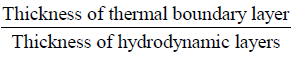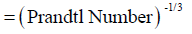Test: Boundary Layer Theory, Laminar Flow - 2 - Question 23

Hydrodynamic and thermal boundary layer thickness is equal forPrandtl number

Detailed Solution for Test: Boundary Layer Theory, Laminar Flow - 2 - Question 23

Ans. (c)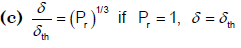Test: Boundary Layer Theory, Laminar Flow - 2 - Question 24

Which non-dimensional number relates the thermal boundary layerand hydrodynamic boundary layer?

Detailed Solution for Test: Boundary Layer Theory, Laminar Flow - 2 - Question 24

Ans. (d) Prandtl number relates the thermal boundary layer and hydrodynamic boundary layer.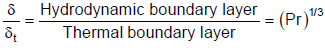Test: Boundary Layer Theory, Laminar Flow - 2 - Question 25

Consider the following statements pertaining to boundary layer:
1. Boundary layer is a thin layer adjacent to the boundary where maximum viscous energy dissipation takes place.
2. Boundary layer thickness is a thickness by which the ideal flow isshifted.
3. Separation of boundary layer is caused by presence of adverse pressure gradient.
Which of these statements are correct?

Detailed Solution for Test: Boundary Layer Theory, Laminar Flow - 2 - Question 25

Ans. (c) 2 is wrong it defines displacement thickness.
Boundary layer thickness: The thickness of the boundary layer is arbitrarily defined as that distance from the boundary in which the velocity reaches 99 percent of the velocity of the free stream. It is denoted by the symbol δ
Displacement thickness: dy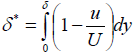It is the distance, measured perpendicular to the boundary, by which the main/free stream is displaced on account of formation of boundary layer.
or,
It is an additional "wall thickness" that would have to be added to compensate
for the reduction in flow rate on account of boundary layer formation

Test: Boundary Layer Theory, Laminar Flow - 2 - Question 26

In a convective heat transfer situation Reynolds number is very largebut the Prandtl number is so small that the product Re × Pr is less thanone in such a condition which one of the following is correct?

Detailed Solution for Test: Boundary Layer Theory, Laminar Flow - 2 - Question 26

Ans. (c)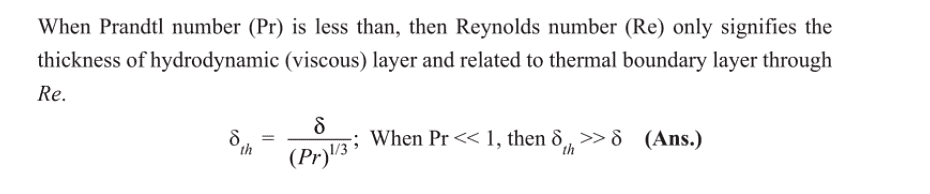Test: Boundary Layer Theory, Laminar Flow - 2 - Question 27

Which one of the following is the correct relationship between the boundary layer thickness δ, displacement thickness δ* and the momentum thickness θ?

Detailed Solution for Test: Boundary Layer Theory, Laminar Flow - 2 - Question 27

Ans. (a) δ >δ*>θ >δ**

Test: Boundary Layer Theory, Laminar Flow - 2 - Question 28

For linear distribution of velocity in the boundary layer on a flat plate,what is the ratio of displacement thickness (δ*) to the boundary layer thickness (δ)?

Detailed Solution for Test: Boundary Layer Theory, Laminar Flow - 2 - Question 28

Ans. (c) Remember it.

Test: Boundary Layer Theory, Laminar Flow - 2 - Question 29

A flat plate, 2m × 0.4m is set parallel to a uniform stream of air (density1.2kg/m3 and viscosity 16 centistokes) with its shorter edges along theflow. The air velocity is 30 km/h. What is the approximate estimated thickness of boundary layer at the down stream end of the plate?

Detailed Solution for Test: Boundary Layer Theory, Laminar Flow - 2 - Question 29

Ans. (b) Thickness of Boundary layer,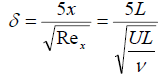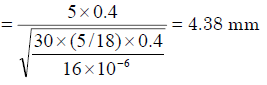Test: Boundary Layer Theory, Laminar Flow - 2 - Question 30

The ‘velocity defect law’ is so named because it governs a

Detailed Solution for Test: Boundary Layer Theory, Laminar Flow - 2 - Question 30

Ans. (c) Figure shows the logarithmic
velocity profile a little away from the wall. Velocity difference (Vmax – V) is known as velocity defect. So velocity defect law occurs due to occurrence of flow with a
logarithmic velocity profile a little away from the wall.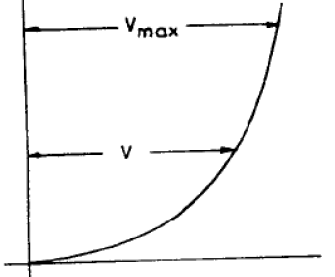## GATE Mechanical (ME) 2023 Mock Test Series

25 docs|229 tests
 Use Code STAYHOME200 and get INR 200 additional OFF Use Coupon Code
Information about Test: Boundary Layer Theory, Laminar Flow - 2 Page
In this test you can find the Exam questions for Test: Boundary Layer Theory, Laminar Flow - 2 solved & explained in the simplest way possible. Besides giving Questions and answers for Test: Boundary Layer Theory, Laminar Flow - 2, EduRev gives you an ample number of Online tests for practice

## GATE Mechanical (ME) 2023 Mock Test Series

25 docs|229 tests